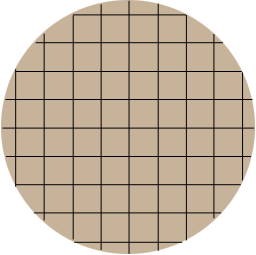SEARCH HOMEMath Central Quandaries & QueriesQuestion from Padmesh, a student: in a circular foundation we are laying a reinforced steel mesh . i.e., like chords in both ways. the circle diameter is 2.91m and rods are placed 0.1m spacing. so I want to find the number of rods present in that circle. here by I am attaching an auto cad drawing for your referenceHi,

Your auto cad drawing didn't arrive but I drew a much smaller situation, similar to what you described. In my situation the diameter of the circle is 4.42 m and the spacing between the rods is 0.5 m.Consider the vertical rods in my drawing. The diameter of the circle is 4.21 m and the spacing between the rods in 0.5 m and $\large \frac{4.21}{0.5} \normalsize = 8.41$ strips. Hence there are 8 strips of width 0.5 m each and a width of 0.41 m which is evenly divided into narrow strips on each side. Thus the rods partition the circle onto 10 strips which requires 9 rods.

I hope this helps,

PennyMath Central is supported by the University of Regina and the Imperial Oil Foundation.